## 4.12Symmetry condition

The transform boundary condition was presented in Sec. 4.11 . It provides a convenient framework for implementing boundary conditions that represent a geometric constraint, including the symmetry condition.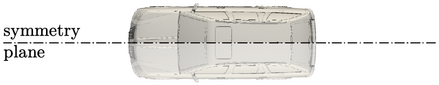The symmetry condition is suitable for simulations where the geometry contains a plane of symmetry and the ﬂow ﬁeld is assumed symmetric. By generating a mesh on one side of a plane of symmetry and applying the symmetry condition, the number of cells, and hence solution time, is reduced.

In the context of a wall boundary, the symmetry condition is also equivalent to slip (as opposed to the common no-slip condition).

A symmetry plane is a transform condition so when the solution variable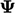is a scalar, it reduces to zero gradient. For a vector, e.g., the condition is zero gradient tangential to the plane, and zero ﬁxed value normal to the plane.

When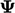is a tensor, the boundary condition requires a more precise deﬁnition, which can also be applied to a vector. The boundary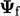can be considered as the mean of the adjacent cell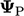and the mirror imagetransformed by the reﬂective transformation tensor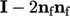, i.e.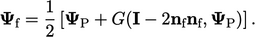(4.18)
Here,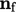is the unit normal vector on the boundary face.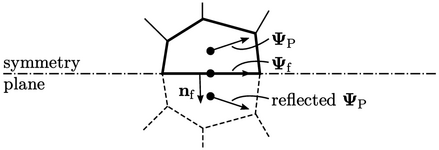Using the notation in Sec. 4.11 , the explicit boundary value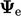is calculated using current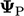from Eq. (4.18 ) and the explicit gradient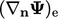is calculated by Eq. (4.17 ).

Comparing Eq. (4.18) with the transform condition of Sec. 4.11 , the factor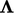corresponds to the tensor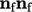. For a vector ﬁeld, a factor which gives good solution convergence is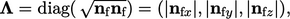(4.19)
where “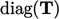” is the vector of the diagonal components of tensor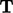.

For a tensor ﬁeld, good convergence is achieved with a tensorcalculated as the outer product offor a vector, i.e. denoting the vector factor by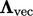, the tensor factor is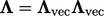.

### Orthogonality condition

The axes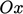,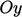,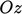, introduced in Sec. 2.1 , must remain orthogonal under a transformation. It requires the transpose of the transformation tensor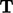to equal its inverse, i.e.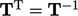.

The orthogonality condition is therefore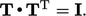The reﬂective transformation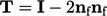satisﬁes the orthogonality condition since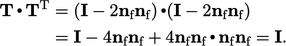Notes on CFD: General Principles - 4.12 Symmetry condition